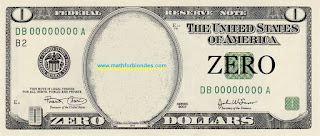## 8.08.2022

### Multiplication by zero and zero element

Mathematicians claim that when multiplied by zero, multiplication occurs and "multiplying by zero (zero element) gives a number equal to zero." Let's look at a couple of examples.

## Zero on the football field

We go to the stadium to watch European football. This is exactly what blondes need. Firstly, beautiful gerls are shown on TV. Secondly, in European football, twenty-two millionaires kick the same ball with varying degrees of success. Where else will blondes find so many rich suitors in one place? And so, the game is in full swing. One player has broken the rules and is removed from the field. What is left on the field instead of this player? An empty space that cannot take part in the game. If the players are numbers, then the empty space is zero. Empty space is not a player, zero is not a number.

Now consider the "null element", which is no different from the "numeric elements". The same football match, the same situation - the player was sent off. And here is the main trick - instead of a remote player, a "zero player" with the number "zero" on a T-shirt enters the field. He joins the game and soon scores a goal. This is where "higher mathematics" begins. One team proves that the zero player is exactly the same as the rest of the players, therefore, has the right to score goals. The other team proves that this is a removed player and he does not have the right to score goals. Here's a great excuse for you to start a "special military operation" like a "football war" between El Salvador and Honduras in 1969. The idiocy of such a situation needs no comment.

## Multiplication by zero in the storeZero dollars

Another example from our life. We all go to the store and buy something. What is the buying process? This is the exchange of the money we have for the goods available in the store. The buying process itself can be compared to multiplication. If the buyer has money, and the store has goods, there are no problems. If the customer doesn't have the money or the store doesn't have the product, then you can't make the purchase. You wouldn’t go to the store with an empty wallet to hear from the seller that you can’t buy anything without money? This situation can be seen as an example of multiplication by zero.

Now consider the process of buying with "zero element". Imagine that you have a bill in your wallet that says "zero dollars". You go to the store with this bill and exchange it for a piece of paper with the inscription "zero goods". Technically, you made a purchase without having a cent in your pocket and without buying anything. Mathematicians tell us about a similar “multiplication by zero (zero element)”.

## Multiplication by zero is not possible

The substitution of concepts can change our logic beyond recognition. This modified logic forces us to look for multiplication by zero where it cannot be - in the results of multiplication. Since multiplication by zero does not occur, then you need to look not into the void (after all, there is no result of multiplication by zero), but into the initial conditions of multiplication. Two apples both lay and will remain lying, even after casting the spell "Apples, I multiply you by zero." Mathematically, this is written to the point of banality simply:

2*0=2*0

All this happens because our mathematicians have not learned to adequately describe reality with the help of mathematics. If you want to look at multiplication examples taken from real life, then you can do it here.

We will consider multiplication by zero in geometry and physics in more detail, to be continued.

P.S. What should you do? Remember that zero is not a number. And when it comes to zero in mathematics, discard your logic and common sense and open the Holy Mathematical Scripture. What is written about the case you are interested in, then tell the mathematicians. You will not begin to assert in a theological seminary that there is no God. So I do not recommend arguing with mathematicians - this is fraught with serious consequences for you. When you become adults and mathematicians disappear from your life, then you can say what you think is right.